# NCERT Solutions for Chapter 10 Mensuration Class 6 MathsChapter Name Mensuration NCERT Solutions Class CBSE Class 6 Textbook Name Maths Related Readings NCERT Solutions for Class 6NCERT Solutions for Class 6 Maths Summary of Mensuration

Exercise 10.1

Question 1: Find the perimeter of each of the following figures: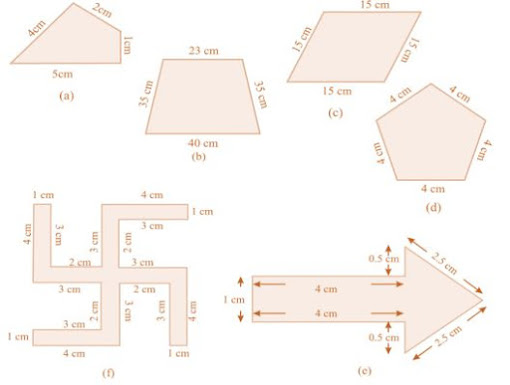Solution

(a) Perimeter = Sum of all the sides

= 4 cm + 2 cm + 1 cm + 5 cm = 12 cm

(b) Perimeter = Sum of all the sides

= 23 cm + 35 cm + 40 cm + 35 cm = 133 cm

(c) Perimeter = Sum of all the sides

= 15 cm + 15 cm + 15 cm + 15 cm = 60 cm

(d) Perimeter = Sum of all the sides

= 4 cm + 4 cm + 4 cm + 4 cm + 4 cm = 20 cm

(e) Perimeter = Sum of all the sides

= 1 cm + 4 cm + 0.5 cm + 2.5 cm + 2.5 cm + 0.5 cm + 4 cm = 15 cm

(f) Perimeter = Sum of all the sides

= 4 cm + 1 cm + 3 cm + 2 cm + 3 cm + 4 cm + 1 cm + 3 cm + 2 cm + 3 cm + 4 cm + 1 cm + 3 cm + 2 cm + 3 cm + 4 cm + 1 cm + 3 cm + 2 cm + 3 cm = 52 cm

Question 2: The lid of a rectangular box of sides 40 cm by 10 cm is sealed all round with tape. What is the length of the tape required?

Solution

Total length of tape required = Perimeter of rectangle

= 2 (40 + 10)

= 2 × 50

= 100 cm

= 1 m

Thus, the total length of tape required is 100 cm or 1 m.

Question 3: A table-top measures 2 m 25 cm by 1 m 50 cm. What is the perimeter of the table-top?

Solution

Length of table top = 2 m 25 cm = 2.25 m

Breadth of table top = 1 m 50 cm = 1.50 m

Perimeter of table top = 2 × (length + breadth)

= 2 × (2.25 + 1.50)

= 2 × 3.75

= 7.50 m

Thus, the perimeter of table top is 7.5 m.

Question 4: What is the length of the wooden strip required to frame a photograph of length and breadth 32 cm and 21 cm respectively?

Solution

Length of wooden strip = Perimeter of photograph

Perimeter of photograph = 2 × (length + breadth)

= 2 (32 + 21)

= 2 × 53 cm

= 106 cm

Thus, the length of the wooden strip required is equal to 106 cm.

Question 5: A rectangular piece of land measures 0.7 km by 0.5 km. Each side is to be fenced with 4 rows of wires. What is the length of the wire needed?

Solution

Since the 4 rows of wires are needed.

Therefore the total length of wires is equal to 4 times the perimeter of rectangle.

Perimeter of field = 2 × (length + breadth)

= 2 × (0.7 + 0.5)

= 2 × 1.2

= 2.4 km

= 2.4 × 1000 m

= 2400 m

Thus, the length of wire = 4 × 2400 = 9600 m = 9.6 km

Question 6: Find the perimeter of each of the following shapes:

(a) A triangle of sides 3 cm, 4 cm and 5 cm.

(b) An equilateral triangle of side 9 cm.

(c) An isosceles triangle with equal sides 8 cm each and third side 6 cm

Solution

(a) Perimeter of ABC

= AB + BC + CA

= 3 cm + 5 cm + 4 cm

= 12 cm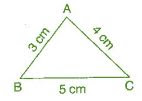(b) Perimeter of equilateral ABC = 3 × side

= 3 × 9 cm

= 27 cm(c) Perimeter of ABC

= AB + BC + CA

= 8 cm + 6 cm + 8 cm

= 22 cm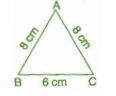Question 7: Find the perimeter of a triangle with sides measuring 10 cm, 14 cm and 15 cm.

Solution

Perimeter of triangle = Sum of all three sides

= 10 cm + 14 cm + 15 cm

= 39 cm

Thus, the perimeter of triangle is 39 cm.

Question 8: Find the perimeter of a regular hexagon with each side measuring 8 cm.

Solution

Perimeter of Hexagon = 6 × length of one side

= 6 × 8 m

= 48 m

Thus, the perimeter of hexagon is 48 m.

Question 9: Find the side of the square whose perimeter is 20 m.

Solution

Perimeter of square = 4 × side

= 20 = 4 × side

= Side = 20/4 = 5 cm

Thus, the side of square is 5 cm.

Question 10: The perimeter of a regular pentagon is 100 cm. How long is its each side?

Solution

Perimeter of regular pentagon = 100 cm

= 5 × side = 100 cm

Side = 100/5 = 20 cm

Thus, the side of regular pentagon is 20 cm.

Question 11: A piece of string is 30 cm long. What will be the length of each side if the string is used to form:

(a) a square

(b) an equilateral triangle

(c) a regular hexagon?

Solution

Length of string = Perimeter of each figure

(a) Perimeter of square = 30 cm

= 4 × side = 30 cm

= Side = 30/4 = 7.5 cm

Thus, the length of each side of square is 7.5 cm.

(b) Perimeter of equilateral triangle = 30 cm

= 3 × side = 30 cm

= Side = 30/3 = 10 cm

Thus, the length of each side of equilateral triangle is 10 cm.

(c) Perimeter of hexagon = 30 cm

= 6 × side = 30 cm

= Side = 30/6 = 5 cm

Thus, the side of each side of hexagon is 5 cm.

Question 12: Two sides of a triangle are 12 cm and 14 cm. The perimeter of the triangle is 36 cm.

What is the third side?

Solution

Let the length of third side be x cm.

Length of other two side are 12 cm and 14 cm.

Now, Perimeter of triangle = 36 cm

=  12+14+x = 36

=  26+x = 36

= x = 36-26

= x = 10 cm

Thus, the length of third side is 10 cm.

Question 13: Find the cost of fencing a square park of side 250 m at the rate of ₹20 per meter.

Solution

Side of square = 250 m

Perimeter of square = 4 × side

= 4 × 250

= 1000 m

Since, cost of fencing of per meter = ₹ 20

Therefore, the cost of fencing of 1000 meters = 20 × 1000 = ₹20,000

Question 14: Find the cost of fencing a rectangular park of length 175 m and breadth 125 m at the rate of ₹12 per meter.

Solution

Length of rectangular park = 175 m

Breadth of rectangular park = 125 m

Perimeter of park = 2 × (length + breadth)

= 2 × (175 + 125)

= 2 × 300 = 600 m

Since, the cost of fencing park per meter = ₹ 12

Therefore, the cost of fencing park of 600 m = 12 × 600 = ₹ 7,200

Question 15: Sweety runs around a square park of side 75 m. Bulbul runs around a rectangular park with length of 60 m and breadth 45 m. Who covers less distance?

Solution

Distance covered by Sweety = Perimeter of square park

Perimeter of square = 4 × side

= 4 × 75 = 300 m

Thus, distance covered by Sweety is 300 m.

Now, distance covered by Bulbul = Perimeter of rectangular park

Perimeter of rectangular park = 2 × (length + breadth)

= 2 × (60 + 45)

= 2 × 105 = 210 m

Thus, Bulbul covers the distance of 210 m and Bulbul covers less distance.

Question 16: What is the perimeter of each of the following figures? What do you infer from the answer?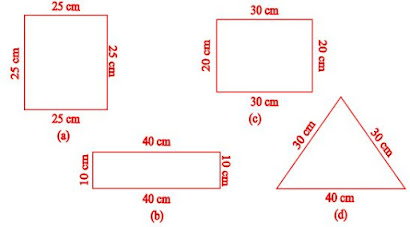Solution

(a) Perimeter of square = 4 × side

= 4 × 25 = 100 cm

(b) Perimeter of rectangle = 2 × (length + breadth)

= 2 × (40 + 10)

= 2 × 50

= 100 cm

(c) Perimeter of rectangle = 2 × (length + breadth)

= 2 × (30 + 20)

= 2 × 50

= 100 cm

(d) Perimeter of triangle = Sum of all sides

= 30 cm + 30 cm + 40 cm

= 100 cm

Thus, all the figures have same perimeter.

Question 17: Avneet buys 9 square paving slabs, each with a side 1/2 m. He lays them in the form of a square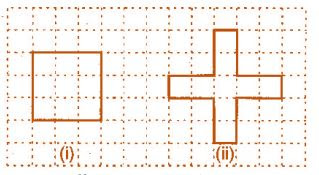(a) What is the perimeter of his arrangement?

(b) Shari does not like his arrangement. She gets him to lay them out like a cross.

What is the perimeter of her arrangement?

(c) Which has greater perimeter?

(d) Avneet wonders, if there is a way of getting an even greater perimeter. Can you find a way of doing this? (The paving slabs must meet along complete edges, i.e., they cannot be broken.)

Solution

(a) 6 m

(b) 10 m

(c) Second arrangement has greater perimeter.

(d) Yes, if all the squares are arranged in row, the perimeter be 10 cm.

Exercise 10.2

Question 1: Find the areas of the following figures by counting squares:Solution

(a) Number of filled square = 9

∴ Area covered by squares = 9 × 1 = 9 sq. units

(b) Number of filled squares = 5

∴ Area covered by filled squares = 5 × 1 = 5 sq. units

(c) Number of full filled squares = 2

Number of half-filled squares = 4

∴ Area covered by full filled squares = 2 × 1 = 2 sq. units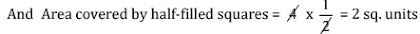∴ Total area = 2 + 2 = 4 sq. units

(d) Number of filled squares = 8

∴ Area covered by filled squares = 8 × 1 = 8 sq. units

(e) Number of filled squares = 10

∴ Area covered by filled squares = 10 × 1 = 10 sq. units

(f) Number of full filled squares = 2

Number of half-filled squares = 4

∴ Area covered by full filled squares = 2 × 1 = 2 sq. units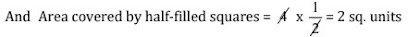∴ Total area = 2 + 2 = 4 sq. units

(g) Number of full filled squares = 4

Number of half-filled squares = 4

∴ Area covered by full filled squares = 4 × 1 = 4 sq. units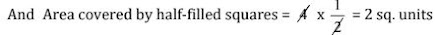∴ Total area = 4 + 2 = 6 sq. units

(h) Number of filled squares = 5

∴ Area covered by filled squares = 5 × 1 = 5 sq. units

(i) Number of filled squares = 9

∴ Area covered by filled squares = 9 × 1 = 9 sq. units

(j) Number of full filled squares = 2

Number of half-filled squares = 4

∴ Area covered by full filled squares = 2 × 1 = 2 sq. units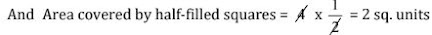∴ Total area = 2 + 2 = 4 sq. units

(k) Number of full filled squares = 4

Number of half-filled squares = 2

∴ Area covered by full filled squares = 4 × 1 = 4 sq. units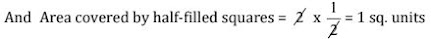∴ Total area = 4 + 1 = 5 sq. units

(l) Number of full filled squares = 3

Number of half-filled squares = 10

∴ Area covered by full filled squares = 3 × 1 = 3 sq. units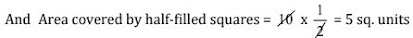∴ Total area = 3 + 5 = 8 sq. units

(m) Number of full filled squares = 7

Number of half-filled squares = 14

∴ Area covered by full filled squares = 7 × 1 = 7 sq. units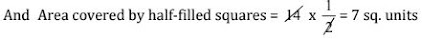∴ Total area = 7 + 7 = 14 sq. units

(n) Number of full filled squares = 10

Number of half-filled squares = 16

∴ Area covered by full filled squares = 10 × 1 = 10 sq. units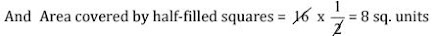∴ Total area = 10 + 8 = 18 sq. units

Exercise 10.3

Question 1: Find the areas of the rectangles whose sides are:

(a) 3 cm and 4 cm

(b) 12 m and 21 m

(c) 2 km and 3 km

(d) 2 m and 70 cm

Solution

(a) Area of rectangle = length × breadth

= 3 cm × 4 cm = 12 cm2

(b) Area of rectangle = length × breadth

= 12 m × 21 m = 252 m2

(c) Area of rectangle = length × breadth

= 2 km × 3 km = 6 km2

(d) Area of rectangle = length × breadth

= 2 m × 70 cm = 2 m × 0.7 m = 1.4 m2

Question 2: Find the areas of the squares whose sides are:

(a) 10 cm

(b) 14 cm

(c) 5 cm

Solution

(a) Area of square = side × side = 10 cm × 10 cm = 100 cm2

(b) Area of square = side × side = 14 cm × 14 cm = 196 cm2

(c) Area of square = side × side = 5 m × 5 m = 25 m2

Question 3: The length and the breadth of three rectangles are as given below:

(a) 9 m and 6 m

(b) 17 m and 3 m

(c) 4 m and 14 m

Which one has the largest area and which one has the smallest?

Solution

(a) Area of rectangle = length × breadth = 9 m × 6 m = 54 m2

(b) Area of rectangle = length × breadth= 3 m × 17 m = 51 m2

(c) Area of rectangle = length × breadth= 4 m × 14 m = 56 m2

Thus, the rectangle (c) has largest area, and rectangle (b) has smallest area.

Question 4: The area of a rectangle garden 50 m long is 300 m2, find the width of the garden.

Solution

Length of rectangle = 50 m and Area of rectangle = 300 m2

Since, Area of rectangle = length × breadth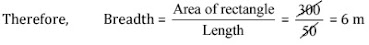Thus, the breadth of the garden is 6 m.

Question 5: What is the cost of tilling a rectangular plot of land 500 m long and 200 m wide at the rate of ₹8 per hundred sq. m?

Solution

Length of land = 500 m and Breadth of land = 200 m

Area of land = length × breadth = 500 m × 200 m = 1,00,000 m2

∵ Cost of tilling 100 sq. m of land = ₹ 8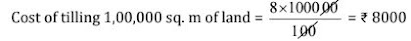Question 6: A table-top measures 2 m by 1 m 50 cm. What is its area in square meters?

Solution

Length of table = 2 m

Breadth of table = 1 m 50 cm = 1.50 m

Area of table = length × breadth

= 2 m × 1.50 m = 3 m2

Question 7: A room us 4 m long and 3 m 50 cm wide. How many square meters of carpet is needed to cover the floor of the room?

Solution

Length of room = 4 m

Breadth of room = 3 m 50 cm = 3.50 m

Area of carpet = length × breadth

= 4 × 3.50 = 14m2

Question 8: A floor is 5 m long and 4 m wide. A square carpet of sides 3 m is laid on the floor. Find the area of the floor that is not carpeted.

Solution

Length of floor = 5 m and breadth of floor = 4 m

Area of floor = length × breadth

= 5 m × 4 m = 20 m2

Now, Side of square carpet = 3 m

Area of square carpet = side × side = 3 × 3 = 9 m2

Area of floor that is not carpeted = 20 m2 – 9 m2 = 11 m2

Question 9: Five square flower beds each of sides 1 m are dug on a piece of land 5 m long and 4 m wide. What is the area of the remaining part of the land?

Solution

Side of square bed = 1 m

∴ Area of square bed = side × side = 1 m × 1 m = 1 m2

Area of 5 square beds = 1 × 5 = 5 m2

Now, Length of land = 5 m

Breadth of land = 4 m

∴ Area of land = length × breadth

= 5 m × 4 m = 20 m2

Area of remaining part = Area of land – Area of 5 flower beds

= 20 m2 – 5 m2 = 15 m2

Question 10: By splitting the following figures into rectangles, find their areas. (The measures are given in centimetres)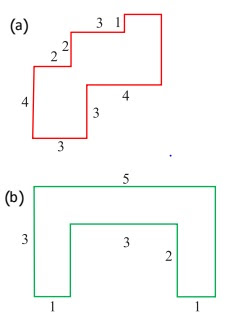Solution

(a) Area of HKLM = 3 × 3 = 9 cm2

Area of IJGH = 1 × 2 = 2 cm2

Area of FEDG = 3 × 3 = 9 cm2

Area of ABCD = 2 × 4 = 8 cm2

Total area of the figure = 9 + 2 + 9 + 8 = 28 cm2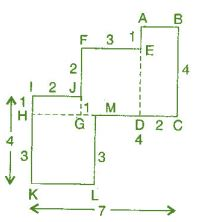(b) Area of ABCD = 3 × 1 = 3 cm2

Area of BDEF = 3 × 1 = 3 cm2

Area of FGHI = 3 × 1 = 3 cm2

Total area of the figure = 3 + 3 + 3 = 9 cm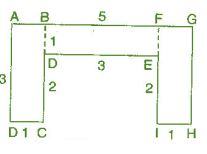Question 11: Split the following shapes into rectangles and find their areas. (The measures are given in centimetres)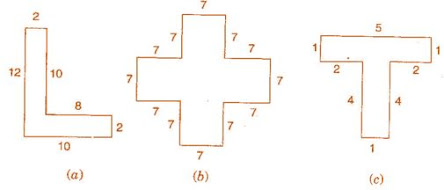Solution

(a) Area of rectangle ABCD = 2 × 10 = 20 cm2

Area of rectangle DEFG = 10 × 2 = 20 cm2

Total area of the figure = 20 + 20 = 40 cm2(b) There are 5 squares each of side 7 cm.

Area of one square = 7 × 7 = 49 cm2

Area of 5 squares = 49 × 5 = 245 cm2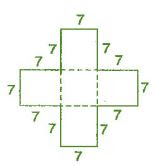(c) Area of rectangle ABCD = 5 × 1 = 5 cm2

Area of rectangle EFGH = 4 × 1 = 4 cm2

Total area of the figure = 5 + 4 cm2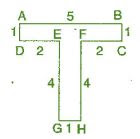Question 12: How many tiles whose length and breadth are 12 cm and 5 cm respectively will be needed

to fit in a rectangular region whose length and breadth are respectively?

(a) 100 cm and 144 cm

(b) 70 cm and 36 cm

Solution

(a) Area of region = 100 cm × 144 cm = 14400 cm2

Area of one tile = 5 cm × 12 cm = 60 cm2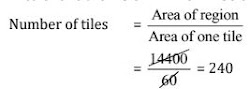Thus, 240 tiles are required.

(b) Area of region = 70 cm × 36 cm = 2520 cm2

Area of one tile = 5 cm × 12 cm = 60 cm2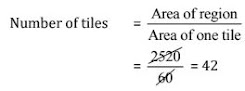Thus, 42 tiles are required.